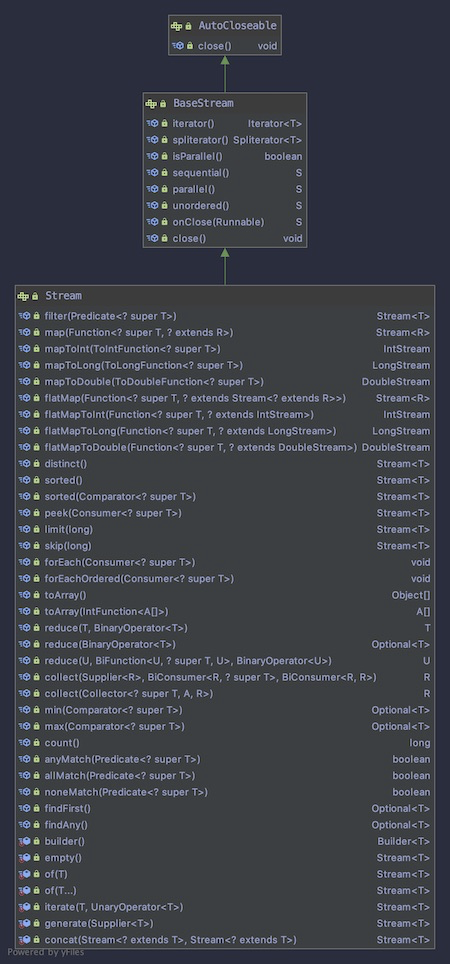# java 8 Streams简介# 创建Stream

Stream的创建有很多方式，java引入Stream之后所有的集合类都添加了一个stream()方法，通过这个方法可以直接得到其对应的Stream。也可以通过Stream.of方法来创建：

``````//Stream Creation
String[] arr = new String[]{"a", "b", "c"};
Stream<String> stream = Arrays.stream(arr);
stream = Stream.of("a", "b", "c");
``````

# Streams多线程

``````//Multi-threading
List<String> list =new ArrayList();
list.parallelStream().forEach(element -> doPrint(element));
``````

# Stream的基本操作

Stream的操作可以分为两类，一类是中间操作，中间操作返回Stream，因此可以级联调用。 另一类是终止操作，这类操作会返回Stream定义的类型。

``````//Operations
long count = list.stream().distinct().count();
``````

## Matching

Stream提供了anyMatch(), allMatch(), noneMatch()这三种match方式，我们看下怎么使用：

``````//Matching
boolean isValid = list.stream().anyMatch(element -> element.contains("h"));
boolean isValidOne = list.stream().allMatch(element -> element.contains("h"));
boolean isValidTwo = list.stream().noneMatch(element -> element.contains("h"));
``````

## Filtering

filter() 方法允许我们对Stream中的数据进行过滤，从而得到我们需要的：

``````Stream<String> filterStream = list.stream().filter(element -> element.contains("d"));
``````

## Mapping

map就是对Stream中的值进行再加工，然后将加工过后的值作为新的Stream返回。

``````//Mapping
Stream<String> mappingStream = list.stream().map(element -> convertElement(element));

private static String convertElement(String element) {
return "element"+"abc";
}
``````

## FlatMap

flatMap和Map很类似，但是他们两个又有不同，看名字我们可以看到flatMap意思是打平之后再做Map。

``````@Data
public class CustBook {

List<String> bookName;
}
``````

CustBook定义了一个bookName字段。

``````List<CustBook> users = new ArrayList<>();
Stream<Stream<String>> userStreamMap
= users.stream().map(user -> user.getBookName().stream());
``````

``````List<CustBook> users = new ArrayList<>();
Stream<String> userStream
= users.stream().map(user -> user.getBookName().stream());
``````

## Reduction

``````//Reduction
List<Integer> integers = Arrays.asList(1, 1, 1);
Integer reduced = integers.stream().reduce(100, (a, b) -> a + b);
``````

## Collecting

collect()方法可以方便的将Stream再次转换为集合类，方便处理和展示：

``````List<String> resultList
= list.stream().map(element -> element.toUpperCase()).collect(Collectors.toList());
``````

# 总结

Stream是java 8中的非常强大和实用的功能，大家一定要熟练掌握。